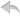注册    登录创作新主题

Python
 python开源   pycharm   Django   Python   DjangoApp
DATA
 docker   Elasticsearch

 闲聊   区块链   招聘   支付之战   翻译   创业   分享发现   分享创造   问与答   求职
WEB开发
 linux   js   其他Web框架   Jquery   peewee   web工具   Git   MongoDB   zookeeper   bottle   Redis   tornado   IE   DATABASE   NoSql   MQ   NGINX   Bootstrap

Python88.com
 反馈   公告   社区推广

Python社区  »  机器学习算法

# 文本分类的机器学习算法

marouane • 2 年前 • 185 次点击
``````def TextClassification(a):

import pandas as pd
#Removing NULL element
#Removing punctuation
df['RÃ©clamation'] = [''.join(c for c in s if c not in string.punctuation) for s in df['RÃ©clamation']]
df['CatÃ©gorie'] = [''.join(c for c in s if c not in string.punctuation) for s in df['CatÃ©gorie']]
#Removing Ã© Ã¨ and all numbers
ch = ['0','1','2','3','4','5','6','7','8','9']

for c in ch:

#Lower case

category_to_id = dict(category_id_df.values)
#library for data preprocessing and maodel building
from sklearn.model_selection import train_test_split
from sklearn.feature_extraction.text import CountVectorizer
from sklearn.feature_extraction.text import TfidfTransformer
from sklearn.svm import LinearSVC
#split data(train/test)
count_vect = CountVectorizer()
X_train_counts = count_vect.fit_transform(X_train)
tfidf_transformer = TfidfTransformer()
X_train_tfidf = tfidf_transformer.fit_transform(X_train_counts)
clf = LinearSVC().fit(X_train_tfidf, y_train)
return(print(clf.predict(count_vect.transform(a)))
``````

`````` File "<ipython-input-25-e6d13da1f205>", line 50
return(print(clf.predict(count_vect.transform(a)))
^
SyntaxError: unexpected EOF while parsing
``````
Python社区是高质量的Python/Django开发社区•   1 楼 Dan D. Yogi    2 年前 你错过了一个 ``` ) ``` ``````return(print(clf.predict(count_vect.transform(a))) ^ ``````